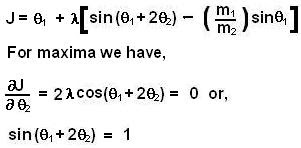## Thursday, June 5, 2008

### Irodov Problem 1.175Suppose that after the collision masses m1 and m2 started moving at anglesanda velocities v1 and v2 respectively as shown in the figure. Since there are no external forces, the momentum before and after collision will be conserved. Since it is an elastic collision initial and final energies will also be conserved. So we have,From (1) and (2) we can obtainFrom (3), (4) and (5) we have,The maximum possible value ofis 1 since its a sine function. This means that the maximum possible value ofis given by,The same result can also be obtained by using Lagrangian multipliers as follows,